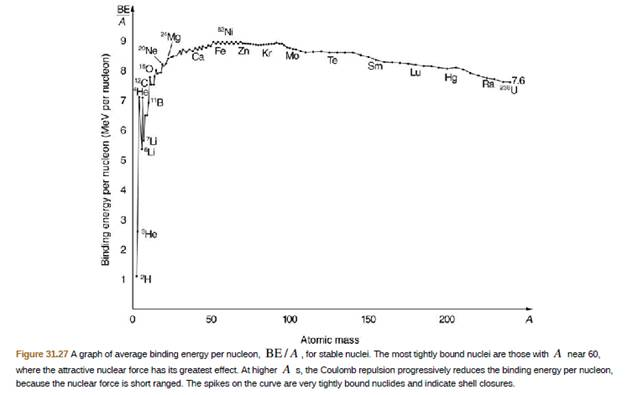Chapter 31, Problem 69PE

Chapter
Section
Textbook Problem

2H is a loosely hound isotope of hydrogen. Called deuterium or heavy hydrogen, it is stable but relatively rare−it is 0.015% of natural hydrogen. Note that deuterium has Z = N, which should tend to make it more tightly bound, but both are odd numbers. Calculate BE/A, the binding energy per nucleon, for 2H and compare it with the approximate value obtained from line graph in Figure 31.27.To determine

The binding energy per nucleon of H2 .

Explanation

Given info:

From periodic table,

The atomic mass A of H2 is 2.014101778u .

Number of protons Z present in H2 is 1 .

The number of neutron present in N

F56e is N=(AZ)=1 .

Formula used:

Formula to find the B.E. per nucleon is,

B.E.A=Z m p+N m nmtotalc2A

Equation-1

Here, mp is the mass of proton, mn is the mass of neutron, mtotal is the total mass and c is the speed of light.

Calculation:

To find the Binding energy per nucleon of H2substitute all the known values in equation-1

B.E.AH2=1× 1

Still sussing out bartleby?

Check out a sample textbook solution.

See a sample solution

The Solution to Your Study Problems

Bartleby provides explanations to thousands of textbook problems written by our experts, many with advanced degrees!

Get Started